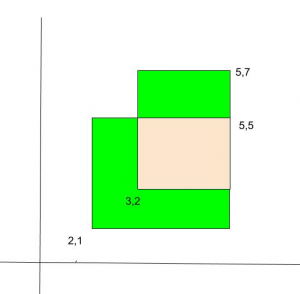# Total area of two overlapping rectangles

• Difficulty Level : Medium
• Last Updated : 13 Jun, 2022

Given two overlapping rectangles on a plane. We are given bottom left and top right points of the two rectangles. We need to find the total area (Green and pink areas in the below diagram).Examples:

```Input : Point l1 = {2, 2}, r1 = {5, 7};
Point l2 = {3, 4}, r2 = {6, 9};
Output :Total Area = 24

Input : Point l1 = {2, 1}, r1 = {5, 5};
Point l2 = {3, 2}, r2 = {5, 7};
Output :Total Area = 16```

We basically add areas of two rectangles. This includes the intersecting part twice, so we subtract the area of intersecting part.

```Total Area = (Area of 1st rectangle +
Area of 2nd rectangle) -
Area of Intersecting part```

Area of Rectangle = x_distance * y_distance

Where,
x_distance for 1st rectangle = abs(l1.x – r1.x)
y_distance for 1st rectangle = abs(l1.y – r1.y)

Similarly, we can compute area of 2nd rectangle.

For area of intersecting part,
x_distance for intersecting rectangle = min(r1.x, r2.x) – max(l1.x, l2.x)
y_distance for 1st rectangle = min(r1.y, r2.y) – max(l1.y, l2.y)

If the x_distance or y_distance is negative, then the two rectangles do not intersect. In that case, overlapping area is 0.

Below is the implementation of the above approach:

## C++

 `// C++ program to find total area of two``// overlapping Rectangles``#include ``using` `namespace` `std;` `struct` `Point {``    ``int` `x, y;``};` `// Returns Total Area  of two overlap``// rectangles``int` `overlappingArea(Point l1, Point r1,``                    ``Point l2, Point r2)``{``    ``// Area of 1st Rectangle``    ``int` `area1 = ``abs``(l1.x - r1.x)``      ``* ``abs``(l1.y - r1.y);` `    ``// Area of 2nd Rectangle``    ``int` `area2 = ``abs``(l2.x - r2.x)``      ``* ``abs``(l2.y - r2.y);` `    ``// Length of intersecting part i.e``    ``// start from max(l1.x, l2.x) of``    ``// x-coordinate and end at min(r1.x,``    ``// r2.x) x-coordinate by subtracting``    ``// start from end we get required``    ``// lengths``    ``int` `x_dist = min(r1.x, r2.x)``                  ``- max(l1.x, l2.x);``    ``int` `y_dist = (min(r1.y, r2.y)``                  ``- max(l1.y, l2.y));``    ``int` `areaI = 0;``    ``if``( x_dist > 0 && y_dist > 0 )``    ``{``        ``areaI = x_dist * y_dist;``    ``}``    ` `    ``return` `(area1 + area2 - areaI);``}` `// Driver Code``int` `main()``{``    ``Point l1 = { 2, 2 }, r1 = { 5, 7 };``    ``Point l2 = { 3, 4 }, r2 = { 6, 9 };` `    ``// Function Call``    ``cout << overlappingArea(l1, r1, l2, r2);``    ``return` `0;``}`

## Java

 `// Java program to find total area of two``// overlapping Rectangles``class` `GFG {` `    ``static` `class` `Point {``        ``int` `x, y;` `        ``public` `Point(``int` `x, ``int` `y)``        ``{``            ``this``.x = x;``            ``this``.y = y;``        ``}``    ``};` `    ``// Returns Total Area of two overlap``    ``// rectangles``    ``static` `int` `overlappingArea(Point l1, Point r1,``                               ``Point l2, Point r2)``    ``{``        ``// Area of 1st Rectangle``        ``int` `area1``            ``= Math.abs(l1.x - r1.x)``              ``* Math.abs(l1.y - r1.y);` `        ``// Area of 2nd Rectangle``        ``int` `area2``            ``= Math.abs(l2.x - r2.x)``              ``* Math.abs(l2.y - r2.y);` `        ``// Length of intersecting part i.e``        ``// start from max(l1.x, l2.x) of``        ``// x-coordinate and end at min(r1.x,``        ``// r2.x) x-coordinate by subtracting``        ``// start from end we get required``        ``// lengths` `        ``int` `x_dist = (Math.min(r1.x, r2.x)``                      ``- Math.max(l1.x, l2.x);``        ``int` `y_dist = (Math.min(r1.y, r2.y)``                 ``- Math.max(l1.y, l2.y);``        ``int` `areaI = ``0``;``        ``if``( x_dist > ``0` `&& y_dist > ``0` `)``        ``{``            ``areaI = x_dist * y_dist;``        ``}` `        ``return` `(area1 + area2 - areaI);``    ``}` `    ``// Driver Code``    ``public` `static` `void` `main(String[] args)``    ``{``        ``Point l1 = ``new` `Point(``2``, ``2``), r1 = ``new` `Point(``5``, ``7``);``        ``Point l2 = ``new` `Point(``3``, ``4``), r2 = ``new` `Point(``6``, ``9``);` `        ``// Function Call``        ``System.out.println(overlappingArea(l1, r1, l2, r2));``    ``}``}` `// This code is contributed by PrinciRaj1992`

## Python3

 `# Python program to find total area of two``# overlapping Rectangles``# Returns Total Area  of two overlap``#  rectangles`  `def` `overlappingArea(l1, r1, l2, r2):``    ``x ``=` `0``    ``y ``=` `1` `    ``# Area of 1st Rectangle``    ``area1 ``=` `abs``(l1[x] ``-` `r1[x]) ``*` `abs``(l1[y] ``-` `r1[y])` `    ``# Area of 2nd Rectangle``    ``area2 ``=` `abs``(l2[x] ``-` `r2[x]) ``*` `abs``(l2[y] ``-` `r2[y])` `    ``''' Length of intersecting part i.e ``        ``start from max(l1[x], l2[x]) of ``        ``x-coordinate and end at min(r1[x],``        ``r2[x]) x-coordinate by subtracting ``        ``start from end we get required ``        ``lengths '''``    ``x_dist ``=` `(``min``(r1[x], r2[x]) ``-``              ``max``(l1[x], l2[x]))` `    ``y_dist ``=` `(``min``(r1[y], r2[y]) ``-``              ``max``(l1[y], l2[y]))``    ``areaI ``=` `0``    ``if` `x_dist > ``0` `and` `y_dist > ``0``:``        ``areaI ``=` `x_dist ``*` `y_dist` `    ``return` `(area1 ``+` `area2 ``-` `areaI)`  `# Driver's Code``l1 ``=` `[``2``, ``2``]``r1 ``=` `[``5``, ``7``]``l2 ``=` `[``3``, ``4``]``r2 ``=` `[``6``, ``9``]` `# Function call``print``(overlappingArea(l1, r1, l2, r2))` `# This code is contributed by Manisha_Ediga`

## C#

 `// C# program to find total area of two``// overlapping Rectangles``using` `System;` `class` `GFG {``    ``public` `class` `Point {``        ``public` `int` `x, y;` `        ``public` `Point(``int` `x, ``int` `y)``        ``{``            ``this``.x = x;``            ``this``.y = y;``        ``}``    ``};` `    ``// Returns Total Area of two overlap``    ``// rectangles``    ``static` `int` `overlappingArea(Point l1, Point r1, Point l2,``                               ``Point r2)``    ``{``        ``// Area of 1st Rectangle``        ``int` `area1``            ``= Math.Abs(l1.x - r1.x) * Math.Abs(l1.y - r1.y);` `        ``// Area of 2nd Rectangle``        ``int` `area2``            ``= Math.Abs(l2.x - r2.x) * Math.Abs(l2.y - r2.y);` `        ``// Length of intersecting part i.e``        ``// start from max(l1.x, l2.x) of``        ``// x-coordinate and end at min(r1.x,``        ``// r2.x) x-coordinate by subtracting``        ``// start from end we get required``        ``// lengths``        ``int` `x_dist``            ``= (Math.Min(r1.x, r2.x) - Math.Max(l1.x, l2.x));``        ``int` `y_dist``            ``= (Math.Min(r1.y, r2.y) - Math.Max(l1.y, l2.y));``        ``int` `areaI = 0;``        ``if` `(x_dist > 0 && y_dist > 0) {``            ``areaI = x_dist * y_dist;``        ``}` `        ``return` `(area1 + area2 - areaI);``    ``}` `    ``// Driver Code``    ``public` `static` `void` `Main(String[] args)``    ``{``        ``Point l1 = ``new` `Point(2, 2), r1 = ``new` `Point(5, 7);``        ``Point l2 = ``new` `Point(3, 4), r2 = ``new` `Point(6, 9);` `        ``// Function Call``        ``Console.WriteLine(overlappingArea(l1, r1, l2, r2));``    ``}``}` `// This code is contributed by PrinciRaj1992`

## Javascript

 ``

Output

`24`

Time complexity: O(1)

Auxiliary Space: O(1)

My Personal Notes arrow_drop_up